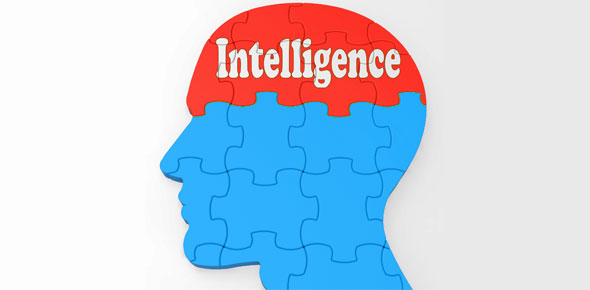# A Tricky IQ Test Questions

9 Questions | Total Attempts: 41Settings• 1.
• A.

• B.

B shows that a is the right answer

• C.

• D.

D all the above is all right

• 2.
If a is b and b is c, m is l what is z ?
• A.

Z is y

• B.

Z is a

• C.

Z is the last letter in alphabet

• D.

Zeeeeee

• 3.
How hot is the sun ?
• A.

The sun is not hot

• B.

I am much more hotter than the sun

• C.

The sun is burning

• D.

Ouch its like 1000 degrees

• 4.
Do you think you got all the three questions correct ?
• A.

No

• B.

Im dumb

• C.

Its impossible to know how many answers i got correct

• D.

Fuck this shit this is jargon

• 5.
Why is there only three options for this question ?
• A.

To decrease the probability of being wrong since this questions is so hard

• B.

Because the creator is too lazy

• C.

To reduce the boring state of the person who is answering this

• 6.
If one plus two is three and four plus two is 6. What is infinity plus infinity
• A.

Infinity power .... i gOT THE POWER

• B.

Infinity squared

• C.

2(infinity)

• 7.
How many blonde does it need to take to make one man to have an erection ?
• A.

Any women will do

• B.

Just one blonde, as long as she is sexy

• C.

200 women !

• 8.
How cold is the hot water ?
• A.

Because of the intensity of the hot water you will feel cold

• B.

Its not that hot

• C.

Its as hot as the cold water

• D.

OUChieee i tried it and its super hot

• 9.
There is only one type of shark that can jump out from the water, what shark is that ?
• A.

This is bullshit

• B.

No shark can jump out of the water

• C.

Tiger shark

• D.

Jumping shark

• E.

Great white shark

Related TopicsBack to top